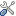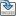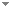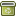# On predicate provability logics and binumerations of fragments of Peano arithmetic

Archive for Mathematical Logic 52 (7-8):871-880 (2013)

 Abstract Solovay proved (Israel J Math 25(3–4):287–304, 1976) that the propositional provability logic of any ∑2-sound recursively enumerable extension of PA is characterized by the propositional modal logic GL. By contrast, Montagna proved in (Notre Dame J Form Log 25(2):179–189, 1984) that predicate provability logics of Peano arithmetic and Bernays–Gödel set theory are different. Moreover, Artemov proved in (Doklady Akademii Nauk SSSR 290(6):1289–1292, 1986) that the predicate provability logic of a theory essentially depends on the choice of a binumeration of the theory which is used to construct the provability predicate. In this paper, we compare predicate provability logics of I∑ n ’s. For a binumeration α(x) of a recursive theory T, let PL α(T) be the predicate provability logic of T defined by α(x). We prove that for any natural numbers i, j such that 0 < i < j, there exists a ∑1 binumeration α(x) of some recursive axiomatization of I∑ i such that ${{\sf PL}_\alpha({\rm I \Sigma}_i) \nsupseteq \bigcap_{\beta(x)}{\sf PL}_\beta({\rm I \Sigma}_j)}$ and ${{\sf PL}_\alpha({\rm I \Sigma}_i) \nsubseteq \bigcup_{\beta(x)}{\sf PL}_\beta({\rm I \Sigma}_j)}$ , where β(x) ranges over all ∑1 binumerations of recursive axiomatizations of I∑ j Keywords Provability logic  Predicate modal logic  Fragments of Peano arithmetic  Binumerations Categories (categorize this paper) ISBN(s) DOI 10.1007/s00153-013-0349-4 OptionsEdit this recordMark as duplicateExport citationFind it on ScholarRequest removal from indexRevision history

PhilArchive copy

Upload a copy of this paper     Check publisher's policy     Papers currently archived: 71,199

Setup an account with your affiliations in order to access resources via your University's proxy server
Configure custom proxy (use this if your affiliation does not provide a proxy)

## References found in this work BETA

The Logic of Provability.George Boolos - 1993 - Cambridge and New York: Cambridge University Press.
The Predicate Modal Logic of Provability.Franco Montagna - 1984 - Notre Dame Journal of Formal Logic 25 (2):179-189.
Metamathematics of First-Order Arithmetic.P. Hájek & P. Pudlák - 2000 - Studia Logica 64 (3):429-430.
No Escape From Vardanyan's Theorem.Albert Visser & Maartje de Jonge - 2006 - Archive for Mathematical Logic 45 (5):539-554.

## Similar books and articles

The Interpretability Logic of Peano Arithmetic.Alessandro Berarducci - 1990 - Journal of Symbolic Logic 55 (3):1059-1089.
Closed Fragments of Provability Logics of Constructive Theories.Albert Visser - 2008 - Journal of Symbolic Logic 73 (3):1081-1096.
On First-Order Theories with Provability Operator.Sergei Artëmov & Franco Montagna - 1994 - Journal of Symbolic Logic 59 (4):1139-1153.
Realization of Intuitionistic Logic by Proof Polynomials.Sergei N. Artemov - 1999 - Journal of Applied Non-Classical Logics 9 (2-3):285-301.
Bimodal Logics for Extensions of Arithmetical Theories.Lev D. Beklemishev - 1996 - Journal of Symbolic Logic 61 (1):91-124.
The Fixed Point Property in Modal Logic.Lorenzo Sacchetti - 2001 - Notre Dame Journal of Formal Logic 42 (2):65-86.
Gödelizing the Yablo Sequence.Cezary Cieśliński & Rafal Urbaniak - 2013 - Journal of Philosophical Logic 42 (5):679-695.